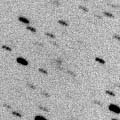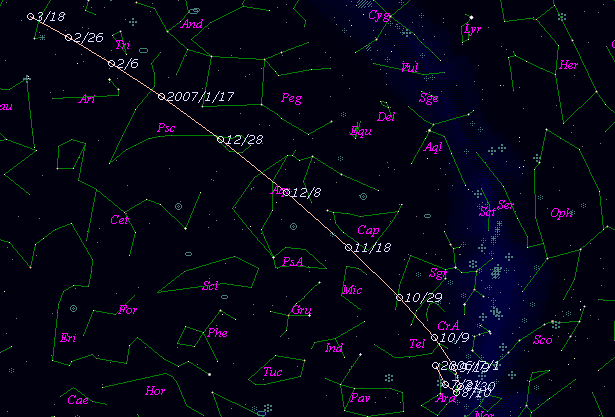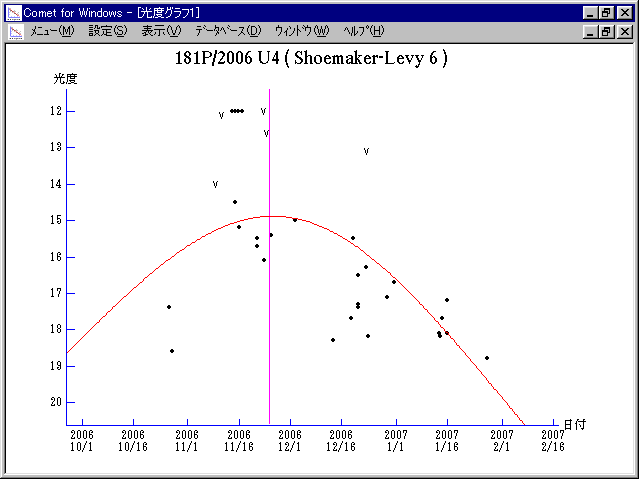# \$B%7%e!<%a!<%+!<(B-\$B%l%t%#Bh#6WB@1(B

181P/Shoemaker-Levy 6 (2006)

 English version Home page Updated on February 12, 2007###\$B%W%m%U%#!<%k(B

 \$BId9f(B 181P/2006 U4 \$B8!=PF|(B 2006\$BG/(B10\$B7n(B26\$BF|(B \$B8!=P8wEY(B 18.2\$BEy(B \$B8!=P R. H. McNaught and D. M. Burton (Siding Spring)

###\$B###\$B50F;MWAG(B

```                    Epoch = 2006 Dec. 11.0 TT
T = 2006 Nov. 25.0013 TT         Peri. = 333.5580
e = 0.706643                     Node  =  37.8728  2000.0
q = 1.127551 AU                  Incl. =  16.9267
a =  3.843617 AU    n = 0.1307959    P =   7.535 years
```

###\$B@1?^(B###\$B8wEYJQ2=(B

```        m1 = 13 + 5 log\$B&\$(B + 40 log r
```##### \$B50F;MWAG\$O(B Dr. Brian G. Marsden \$B\$N7W;;\$K\$h\$k\$b\$N\$G!"(B IAUC 8767 \$B\$K7G:\\$5\$l\$?\$b\$N\$G\$9!#(B \$B@1?^\$O(B StellaNavigator Ver.2.0 for Windows (\$B%"%9%H%m%"!<%D(B \$BJTCx(B / \$B%"%9%-!<=PHG6I4)(B) \$B\$G:n@.\$7\$?\$b\$N\$G\$9!#(B \$B8wEY%0%i%U\$O(BComet for Windows\$B\$G:n@.\$7\$?\$b\$N\$G\$9!#(BCopyright(C) Seiichi Yoshida (comet@aerith.net). All rights reserved.